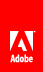Comparing strings

Flash Player 9 and later, Adobe AIR 1.0 and later

 You can use the following operators to compare strings: < , <= , != , == , => , and > . These operators can be used with conditional statements, such as if and while , as the following example shows: var str1:String = "Apple"; var str2:String = "apple"; if (str1 < str2) { trace("A < a, B < b, C < c, ..."); } When using these operators with strings, ActionScript considers the character code value of each character in the string, comparing characters from left to right, as in the following: trace("A" < "B"); // true trace("A" < "a"); // true trace("Ab" < "az"); // true trace("abc" < "abza"); // true Use the == and != operators to compare strings with each other and to compare strings with other types of objects, as the following example shows: var str1:String = "1"; var str1b:String = "1"; var str2:String = "2"; trace(str1 == str1b); // true trace(str1 == str2); // false var total:uint = 1; trace(str1 == total); // trueTwitter™ and Facebook posts are not covered under the terms of Creative Commons.// Ethnio survey code removed